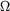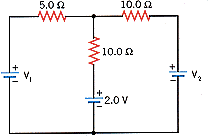Advanced Electricity and Magnetism Advanced Electricity and Magnetism Physics Help ForumFeb 6th 2009, 11:51 AM #1 Junior Member   Join Date: Feb 2009 Posts: 1 Circuit Determine the voltage across the 5.0resistor in the drawing (V1 = 12.0 V and V2 = 16.0 V). 1 V Which end of the resistor is at the higher potential? 2 left end of the resistor right end of the resistorSection 20.10 Kirchhoff's RulesFeb 6th 2009, 10:08 PM #2 Senior Member   Join Date: Dec 2008 Location: Las Cruces NM Posts: 256 I notice that your problem is similar to the circuit worked on the page: Examples In terms of the variables in that problem $\displaystyle V_a = 10$ $\displaystyle V_b = 14$ to allow for the effect of the 2 volt battery. You would have to solve the simultaneous eq 4 & 5 on that page without assuming all resistors are the same. (I dont know why that page introduced that assumption.)Feb 7th 2009, 07:34 AM #3 Banned   Join Date: Jan 2009 Posts: 21 You can use loop $\displaystyle R = 5 \ohm$ $\displaystyle V = 2.0 V$ For left loop: $\displaystyle V_1 - V = I_1 R + (I_1 + I_2) 2R$ Right loop: $\displaystyle V_2 - V = I_2 2R + (I_1+I_2) 2R$ Solve the equations for currents, and then you can determine which resistor has greater voltage.Tags circuitThread ToolsShow Printable VersionEmail this Page Display ModesLinear ModeSwitch to Hybrid ModeSwitch to Threaded ModeSimilar Physics Forum Discussions Thread Thread Starter Forum Replies Last Post rcmango Electricity and Magnetism 0 Aug 16th 2011 01:40 AM missyc8 Electricity and Magnetism 3 Sep 30th 2009 09:19 AM helloying Electricity and Magnetism 2 Apr 15th 2009 04:08 AM tribble1 Advanced Electricity and Magnetism 1 Mar 22nd 2009 08:18 PM karnold9 Advanced Electricity and Magnetism 3 Sep 26th 2008 07:50 PM